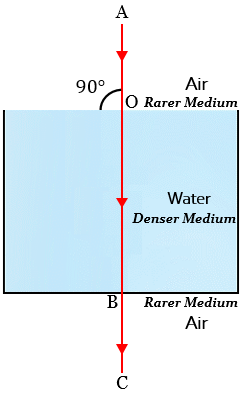# A vertical ray of light strikes the horizontal surface of some water:(a) What is the angle of incidence?(b) What is the angle of refraction?

(a) Angle of incidence is  because the light ray is striking vertically (along the normal) to the horizontal surface of some water and the angle of incidence is the angle between the light ray and the normal.

(b) Angle of refraction is also because when the incident ray falls vertically on the surface, no bending of light ray takes place.

Explanation

If the light ray hits the water at right angles (at 90°) to the surface, then the light ray will not bend (no refraction).Updated on: 10-Oct-2022

258 Views